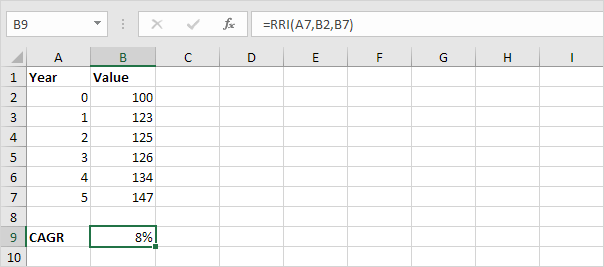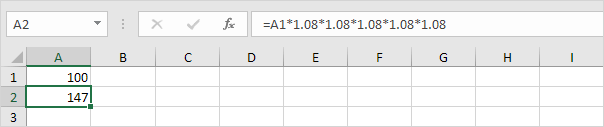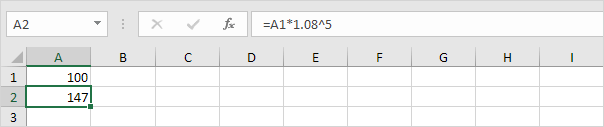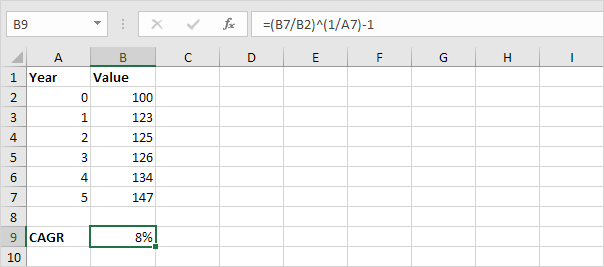# CAGR

There's no CAGR function in Excel. However, simply use the RRI function in Excel to calculate the compound annual growth rate (CAGR) of an investment over a period of years.

1. The RRI function below calculates the CAGR of an investment. The answer is 8%.Note: the RRI function has three arguments (number of years = 5, start = 100, end = 147).

2. The CAGR measures the growth of an investment as if it had grown at a steady rate on an annually compounded basis. We can check this.which is the same as:Note: again, number of years or n = 5, start = 100, end = 147, CAGR = 8%.

3. Knowing this, we can easily create a CAGR formula that calculates the compound annual growth rate of an investment in Excel.

A2 = A1 * (1 + CAGR)n

end = start * (1 + CAGR)n

end/start = (1 + CAGR)n

(end/start)1/n = (1 + CAGR)

CAGR = (end/start)1/n - 1

4. The CAGR formula below does the trick.Note: in other words, to calculate the CAGR of an investment in Excel, divide the value of the investment at the end by the value of the investment at the start. Next, raise this result to the power of 1 divided by the number of years. Finally, subtract 1 from this result.

Go to Next Chapter: Statistical Functions